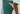# 3 Easy Methods for Converting Fractions and Decimals

POSTED ON FEBRUARY 22, 2023Fractions and decimals, decimals and fractions- two tricky little math concepts that have given many young math geniuses frustration. Both decimals and fractions are different ways of expressing the same thing. They name parts of a whole.

A fraction labels the parts of a whole with a numerator (top number) and a denominator (bottom number), like the examples below.A decimal names part of a whole with a dot called a decimal point and place value positions- tenths, hundredths, thousandths, and on and on.Since fractions and decimals both represent parts of a whole number, they can be used interchangeably. A fraction can be converted into a decimal, and a decimal can be converted to a fraction. Depending on the math problem or numbers involved, there are different methods, or ways, to make the conversion and make the numbers work for you.

### Method #1- Basic Conversions

The first method is simple and uses basic place value skills. In a place value chart, the numbers after the decimal point have names that correspond to the denominator in some fractions.The number in the chart above is written as 0.325. When said out loud, or written in word form, the fraction name is easy to hear.When converting this decimal to it’s fraction equivalent, the place value tells us the denominator and the digits tell us the denominator.Here are more examples of this basic method to convert fractions to decimals or decimals to fractions.Boost math confidence to the next level
Juni’s vetted instructors study at top US Universities and provide our students with the support and mentorship to grow their math skills.

### Method #2- Equivalent Fractions

The trouble with the first method of converting fractions and decimals is that it only works if the denominator in the fraction is 10, 100, 1,000, etc.

For example, the fraction below has a denominator of 25. Twenty-fifths is not a number that exists in decimal place value. This means, there is another step to converting this fraction to a decimal- finding an equivalent fraction.In this case, you can multiply the denominator 25 by 4 to turn it into 100, which can then be easily converted into a decimal. However, there is one VERY important rule when making equivalent fractions. Whatever you do to the denominator, you must also do to the numerator.Try this method out on the fractions below:Our advisors can answer any questions you have about our curriculum or subscriptions. They can even provide a course recommendation.

### Method #3- Long Division

Even though the first and second method of converting fractions to decimals are fairly easy, they don’t work in every case. Some fractions just don’t have an equivalent that is easy to convert. That leaves one final method for converting fractions to decimals- long division. Simply divide the digits in the fraction and move the decimal into the right position, and you will have the decimal equivalent.

Converting fractions to decimals or decimals to fractions can be done quickly and easily with the right method. Give each process a try and see which works best for the math problem on hand.

Juni Learning has excellent resources for exploring the new landscape of math learning and comprehension. If you’d like to encourage more interaction with lessons in Common Core’s mathematical standards, visit Juni Learning to explore their options. Just like those math standards, Juni Learning’s catalog can encourage exploration in other subjects, too!

Juni Learning is also launching new tutoring help. The Juni Tutor Bot is an AI-powered tutoring solution designed to provide personalized help to students for homework help and test preparation. The bot is available on Discord, a popular communication platform, and can be accessed by signing up for the Juni Tutor Bot. Once signed up, students can chat with the Juni Tutor Bot and receive customized help based on their unique learning needs.